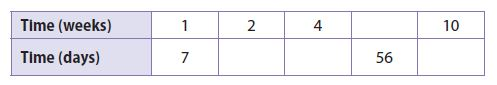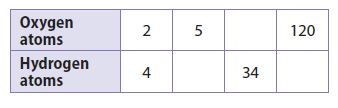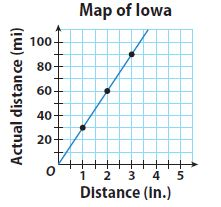Homework Explained - Math Practice 101Dear guest, you are not a registered member. As a guest, you only have read-only access to our books, tests and other practice materials.

As a registered member you can:

Registration is free and doesn't require any type of payment information. Click here to Register.
Go to page:
Chapter 3: Proportional Relationships; Lesson 1: Representing Proportional Relationships

Guided Practice

• Question 1

Vocabulary

A proportional relationship is a relationship between two quantities in which the ratio of one quantity to the other quantity is/is not constant.

•  constant
• Question 2

Vocabulary

When writing an equation of a proportional relationship in the form $$\text{y} = \text{kx}$$, k represents the __________________________.

• Question 3

Write an equation that describes the proportional relationship between the number of days and the number of weeks in a given length of time.

a. Complete the table.• Type below:
• Question 3

b. Let x represent _____.
Let y represent _____.
The equation that describes the relationship is _____.

• Type below:

Each table or graph represents a proportional relationship. Write an equation that describes the relationship.

• Question 4

Physical Science

The relationship between the numbers of oxygen atoms and hydrogen atoms in water.• Type below:
• Question 5• Type below:

Essential Question Check-In

• Question 6

If you know the equation of a proportional relationship, how can you draw the graph of the equation?

• Type below:

Yes, email page to my online tutor. (if you didn't add a tutor yet, you can add one here)